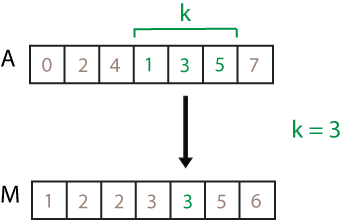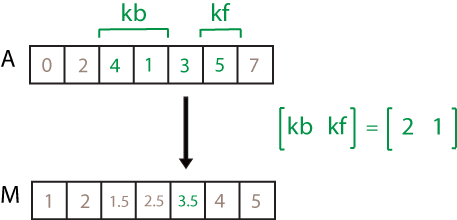# movmedian

## Syntax

``M = movmedian(A,k)``
``````M = movmedian(A,[kb kf])``````
``M = movmedian(___,dim)``
``M = movmedian(___,nanflag)``
``M = movmedian(___,Name,Value)``

## Description

example

````M = movmedian(A,k)` returns an array of local `k`-point median values, where each median is calculated over a sliding window of length `k` across neighboring elements of `A`. When `k` is odd, the window is centered about the element in the current position. When `k` is even, the window is centered about the current and previous elements. The window size is automatically truncated at the endpoints when there are not enough elements to fill the window. When the window is truncated, the median is taken over only the elements that fill the window. `M` is the same size as `A`.If `A` is a vector, then `movmedian` operates along the length of the vector.If `A` is a multidimensional array, then `movmedian` operates along the first array dimension whose size does not equal 1.```

example

``````M = movmedian(A,[kb kf])``` computes the median with a window of length `kb+kf+1` that includes the element in the current position, `kb` elements backward, and `kf` elements forward.```

example

````M = movmedian(___,dim)` returns the array of moving medians along dimension `dim` for any of the previous syntaxes. For example, if `A` is a matrix, then `movmedian(A,k,2)` operates along the columns of `A`, computing the `k`-element sliding median for each row.```

example

````M = movmedian(___,nanflag)` specifies whether to include or omit `NaN` values from the calculation for any of the previous syntaxes. `movmedian(A,k,'includenan')` includes all `NaN` values in the calculation while `movmedian(A,k,'omitnan')` ignores them and computes the median over fewer points.```

example

````M = movmedian(___,Name,Value)` specifies additional parameters for the moving median using one or more name-value pair arguments. For example, if `x` is a vector of time values, then `movmedian(A,k,'SamplePoints',x)` computes the moving median relative to the times in `x`.```

## Examples

collapse all

Compute the three-point centered moving median of a row vector. When there are fewer than three elements in the window at the endpoints, take the average over the elements that are available.

```A = [4 8 6 -1 -2 -3 -1 3 4 5]; M = movmedian(A,3)```
```M = 1×10 6.0000 6.0000 6.0000 -1.0000 -2.0000 -2.0000 -1.0000 3.0000 4.0000 4.5000 ```

Compute the three-point trailing moving median of a row vector. When there are fewer than three elements in the window at the endpoints, take the average over the elements that are available.

```A = [4 8 6 -1 -2 -3 -1 3 4 5]; M = movmedian(A,[2 0])```
```M = 1×10 4 6 6 6 -1 -2 -2 -1 3 4 ```

Compute the three-point centered moving median for each row of a matrix. The window starts on the first row, slides horizontally to the end of the row, then moves to the second row, and so on. The dimension argument is two, which slides the window across the columns of `A`.

`A = [4 8 6; -1 -2 -3; -1 3 4]`
```A = 3×3 4 8 6 -1 -2 -3 -1 3 4 ```
`M = movmedian(A,3,2)`
```M = 3×3 6.0000 6.0000 7.0000 -1.5000 -2.0000 -2.5000 1.0000 3.0000 3.5000 ```

Compute the three-point centered moving median of a row vector containing `NaN` elements.

```A = [4 8 NaN -1 -2 -3 NaN 3 4 5]; M = movmedian(A,3)```
```M = 1×10 6.0000 NaN NaN NaN -2.0000 NaN NaN NaN 4.0000 4.5000 ```

Recalculate the median, but omit the `NaN` values. When `movmedian` discards `NaN` elements, it takes the median over the remaining elements in the window.

`M = movmedian(A,3,'omitnan')`
```M = 1×10 6.0000 6.0000 3.5000 -1.5000 -2.0000 -2.5000 0 3.5000 4.0000 4.5000 ```

Compute a 3-hour centered moving median of the data in `A` according to the time vector `t`.

```A = [4 8 6 -1 -2 -3]; k = hours(3); t = datetime(2016,1,1,0,0,0) + hours(0:5)```
```t = 1x6 datetime Columns 1 through 3 01-Jan-2016 00:00:00 01-Jan-2016 01:00:00 01-Jan-2016 02:00:00 Columns 4 through 6 01-Jan-2016 03:00:00 01-Jan-2016 04:00:00 01-Jan-2016 05:00:00 ```
`M = movmedian(A,k,'SamplePoints',t)`
```M = 1×6 6.0000 6.0000 6.0000 -1.0000 -2.0000 -2.5000 ```

Compute the three-point centered moving median of a row vector, but discard any calculation that uses fewer than three points from the output. In other words, return only the medians computed from a full three-element window, discarding endpoint calculations.

```A = [4 8 6 -1 -2 -3 -1 3 4 5]; M = movmedian(A,3,'Endpoints','discard')```
```M = 1×8 6 6 -1 -2 -2 -1 3 4 ```

## Input Arguments

collapse all

Input array, specified as a vector, matrix, or multidimensional array.

Data Types: `single` | `double` | `int8` | `int16` | `int32` | `int64` | `uint8` | `uint16` | `uint32` | `uint64` | `logical`

Window length, specified as a numeric or duration scalar. When `k` is a positive integer scalar, the centered median includes the element in the current position plus surrounding neighbors. For example, a three-point median defined by a window of length three results in the following calculation for a vector `A`:Data Types: `single` | `double` | `int8` | `int16` | `int32` | `int64` | `uint8` | `uint16` | `uint32` | `uint64` | `duration`

Directional window length, specified as a numeric or duration row vector containing two elements. When `kb` and `kf` are positive integer scalars, the calculation is over `kb+kf+1` elements. The calculation includes the element in the current position, `kb` elements before the current position, and `kf` elements after the current position. For example, a four-point median defined by the directional window `[2 1]` results in the following calculation for a vector `A`:Data Types: `single` | `double` | `int8` | `int16` | `int32` | `int64` | `uint8` | `uint16` | `uint32` | `uint64` | `duration`

Dimension to operate along, specified as a positive integer scalar. If no value is specified, then the default is the first array dimension whose size does not equal 1.

Dimension `dim` indicates the dimension that `movmedian` operates along, that is, the direction in which the specified window slides.

Consider a two-dimensional input array, `A`.

• If `dim = 1`, then `movmedian(A,k,1)` starts with the first column and slides vertically over each row. The median is computed over `k` elements at a time. Then it moves to the second column and repeats the computation. This process continues until all columns are exhausted.• If `dim = 2`, then `movmedian(A,k,2)` starts with the first row and slides horizontally across each column. The median is computed over `k` elements at a time. Then it moves to the second row and repeats the computation. This process continues until all rows are exhausted.Data Types: `double` | `single` | `int8` | `int16` | `int32` | `int64` | `uint8` | `uint16` | `uint32` | `uint64`

`NaN` condition, specified as one of these values:

• `'includenan'` — Include `NaN` values from the input when computing the median, resulting in the output `NaN`.

• `'omitnan'` — Ignore all `NaN` values in the input. If a window contains only `NaN` values, then `movmedian` returns `NaN`.

### Name-Value Pair Arguments

Specify optional comma-separated pairs of `Name,Value` arguments. `Name` is the argument name and `Value` is the corresponding value. `Name` must appear inside quotes. You can specify several name and value pair arguments in any order as `Name1,Value1,...,NameN,ValueN`.

Example: `M = movmedian(A,k,'Endpoints','fill')`

Method to treat leading and trailing windows, specified as the comma-separated pair consisting of `'Endpoints'` and one of the following:

`'Endpoints'` ValueDescription
`'shrink'`Shrink the window size near the endpoints of the input to include only existing elements.
`'discard'`Do not output any medians when the window does not completely overlap with existing elements.
`'fill'`Substitute nonexisting elements with `NaN`.
numeric or logical scalarSubstitute nonexisting elements with a specified numeric or logical value.

Data Types: `double` | `single` | `int8` | `int16` | `int32` | `int64` | `uint8` | `uint16` | `uint32` | `uint64` | `logical` | `char` | `string`

Sample points for computing medians, specified as the comma-separated pair consisting of `'SamplePoints'` and a vector. The sample points represent the location of the data in `A`. Sample points do not need to be uniformly sampled. By default, the sample points vector is `[1 2 3 ... ]`.

Moving windows are defined relative to the sample points, which must be sorted and contain unique elements. For example, if `t` is a vector of times corresponding to the input data, then `movmedian(rand(1,10),3,'SamplePoints',t)` has a window that represents the time interval between `t(i)-1.5` and `t(i)+1.5`.

When the sample points vector has data type `datetime` or `duration`, then the moving window length must have type `duration`.

If the sample points are nonuniformly spaced and the `'Endpoints'` name-value pair is specified, then its value must be `'shrink'`.

Data Types: `single` | `double` | `int8` | `int16` | `int32` | `int64` | `uint8` | `uint16` | `uint32` | `uint64` | `datetime` | `duration`